# System Identification

In GPC (Global Predictive Control) controllers

, we must simulate the process to control. To preform the simulation we must first identify the system. There are mainly three type of identification algorithms: global, structured and local.

## Global identification

We start from the data (see fig.1 below):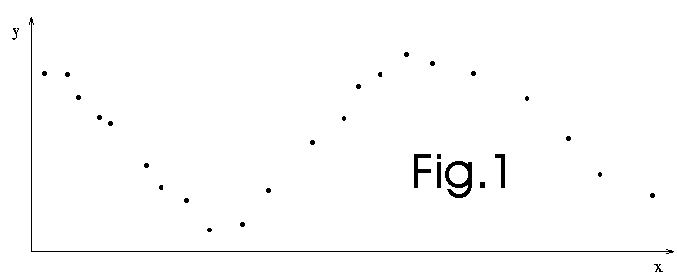We use all the datas available to construct a global model (see fig. 2 below). This technique is used, for example, in artificial neural network (NN) regressors.We discard the data. To make the prediction, we now only use the model (see fig. 3 below):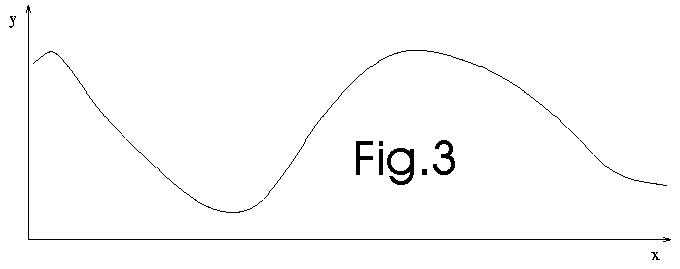This technique is used, for example, in artificial neural network (NN) regressors. The NN is trained (often using gradient backpropagation) to fit the whole dataset.

## Structural identification.

We, once again, start from the data (see fig 1 below):We perform a series of local fitting (see fig. 4 below). This technique is used, for example, in Takagi-Sugeno fuzzy systems. Each rule of these systems correspond to a part of the space. At each rule, there is a local model tailored for this small part of the space. Thus, in the example below, there are 3 rules. The quality of the prediction hardly depends on the number of rules. In the NLMIMO toolbox, we use 10-fold cross-validation technique to find the optimal number of rules. It's a very long process.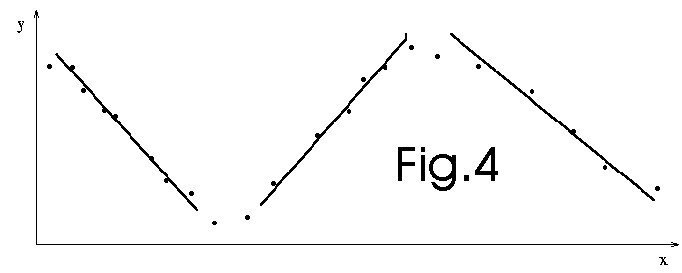We discard the data. To make the prediction, we now only use the models (see fig. 5 below):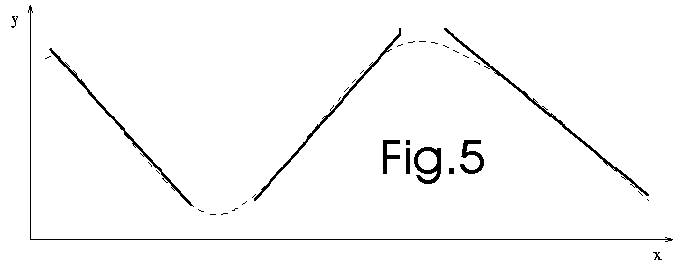## Local identification: The Lazy Learning approach.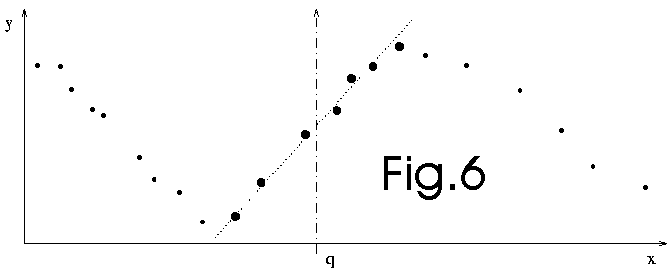Lazy learning postpones all the computation until an explicit request for a prediction is received. The request is fulfilled by modelling locally the relevant data stored in the database and using the newly constructed model to answer the request. Each prediction requires therefore a local modelling procedure. We will use, as regressor, a simple polynomial. The number of points used for the construction of this polynomial (in other words, the kernel size or the bandwidth) and the degree of the polynomial (0,1, or 2) are chosen using leave-one out cross-validation technique. The identification of the polynomial is performed using a recursive least square algorithm. The cross-validation is performed at high speed using PRESS statistics.

What is leave-one out cross-validation? This is a technique used to evaluate to quality of a regressor build on a dataset of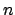points. First, take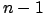points from the dataset (These points are the training set). The last point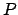will be used for validation purposes (validation set). Build the regressor with the training set. Use this new regressor to predict the value of the only pointwhich has not been used during the building process. You obtain a fitting error between the true value ofand the predicted one. Continue, in a similar way, to build regressors, each time on a different dataset ofpoints. You obtainfitting errors for theregressors you have build. The global cross-validation error is the mean of all thesefitting errors.

We will choose the polynomial which has the lowest cross-validation error. We can also give an estimate of the quality of the prediction: it's simply the cross-validation error of the chosen model.

We can also choose the k polynomials which have the lowest cross-validation error and use, as final model, a new model which is the weighted average of these k polynomials. The weight is the inverse of the cross-validation error. In the Lazy learning toolbox, other possibilities of combination are possible.

The Lazy Learning requires a dataset in which the variance of the noise in the data is locally constant. There can be a huge noise on a small part of the data and nearly no noise everywhere else: This is no problem. On the contrary, global identification tools like Neural Networks demands that the variance of the noise in the data is globally constant. This is why Lazy Learning performs nearly systematically better than other classical global identification techniques.

Another advantage is the facility for on-line adaptation of the regressor. In the case of Takagi-Sugeno fuzzy systems, each time we increase the training set we have to wait several minutes for the computation of the new optimal number of rules, the calculation of the new "places" for each rules in the space and the construction of all the local models. On the contrary, in the case of Lazy Learning, we only have to add one point inside the training dataset. That's all. You can see here an example of on-line adaptation with lazy learning.

One drawback of the lazzy learning approach is that it takes time to construct at each query a good local model. Fortunately, most of the calculation time is spend in the search of the neighbours points inside the dataset. For a small dataset (less than 10000 points), the query is fullfilled immediatly (no wait time on a P3 600 Mhz) (the search-loop has been highly optimized).

## What to use and when ?

Suppose you want to identify some datas. What tool is the best for the job?

## Bibliography

The excellent book of Myers "Classical and Modern Regression With Applications" contains all the mathematical demonstration used for the recursive least square and the Press statistics.

For the implementation of these algorithms inside the Lazy Learning toolbox:
- "M. Birattari, G. Bontempi, and H. Bersini. Lazy learning meets the recursive least-squares algorithm. In M. S. Kearns, S. A. Solla, and D. A. Cohn, editors, Advances in Neural Information Processing Systems 11, Cambridge, 1999. MIT Press.", available here.
- "Gianluca Bontempi and Mauro Birattari and Hugues Bersini, Recursive Lazy Learning for Modeling and Control in "European Conference on Machine Learning", pp 292-303, 1998", available here.